# Delta to Wye Conversion

Created by Bogna Szyk and Kenneth Alambra
Reviewed by Dominik Czernia, PhD candidate and Jack Bowater
Last updated: Mar 07, 2022

This delta to wye conversion will help you transform a delta network of resistors to a wye network (or 'Y" network) and vice versa. In this calculator, you will learn the difference between delta and wye networks of resistors, the formulas for delta to wye conversion, how to convert delta to wye networks, and some applications of delta and wye networks of resistors.

Keep on reading to learn how to transform a complicated electronic circuit with wye and delta diagrams into a simple one (with only parallel and series-connected resistors) using the delta to "Y" conversion.

## Delta vs. wye network of resistors

In electronic circuits, the resistor (shown in the image below) is one of the most important components and has a wide variety of uses. We can use resistors to limit the current in a circuit or the voltage flowing towards another component of the circuit.Since circuits can have multiple components in them, like capacitors, inductors, LEDs, motors, and so much more, circuits can also have lots of resistors in them. Resistors in circuits usually connected either in parallel or in series but can sometimes form loops and multiple-branch networks, which are seen in more complicated circuits. The simplest loop that resistors can make is the delta network, as shown in the diagram below: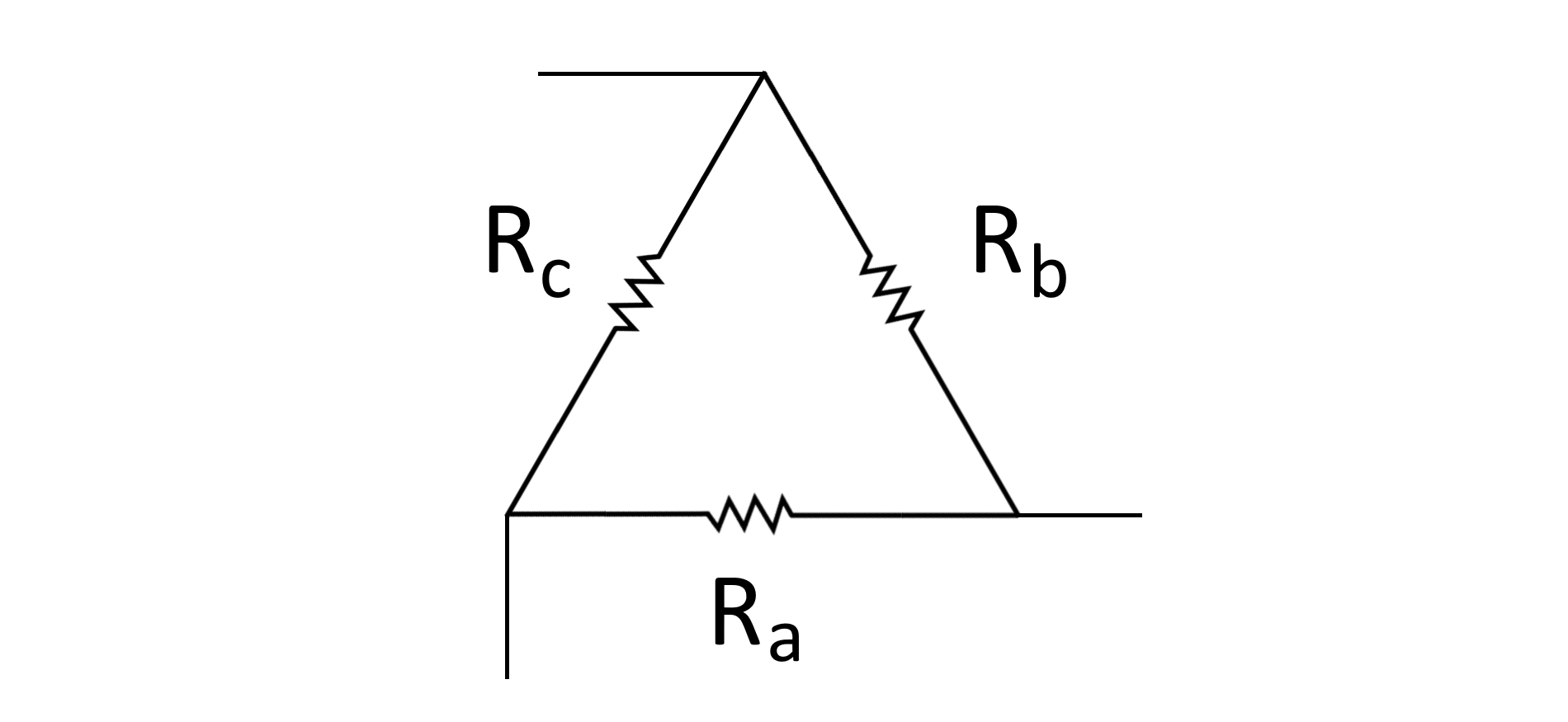In electronics diagrams, a resistor is usually represented by a zigzag line. In a delta network, we connect three resistors to three nodes (represented by A, B, and C) that lead to three different parts of a circuit. We call it a "delta" network because, in its simplest diagrammatic representation, this network forms a triangular shape just like the uppercase Greek symbol delta Δ.

The difference between a delta and a wye network is that, in a wye network, we connect the said three nodes in a different fashion by extending the three nodes using three resistors and connecting all these resistors to a single node. This forms something like a letter Y, hence the name "wye" network. Here is what a wye network looks like: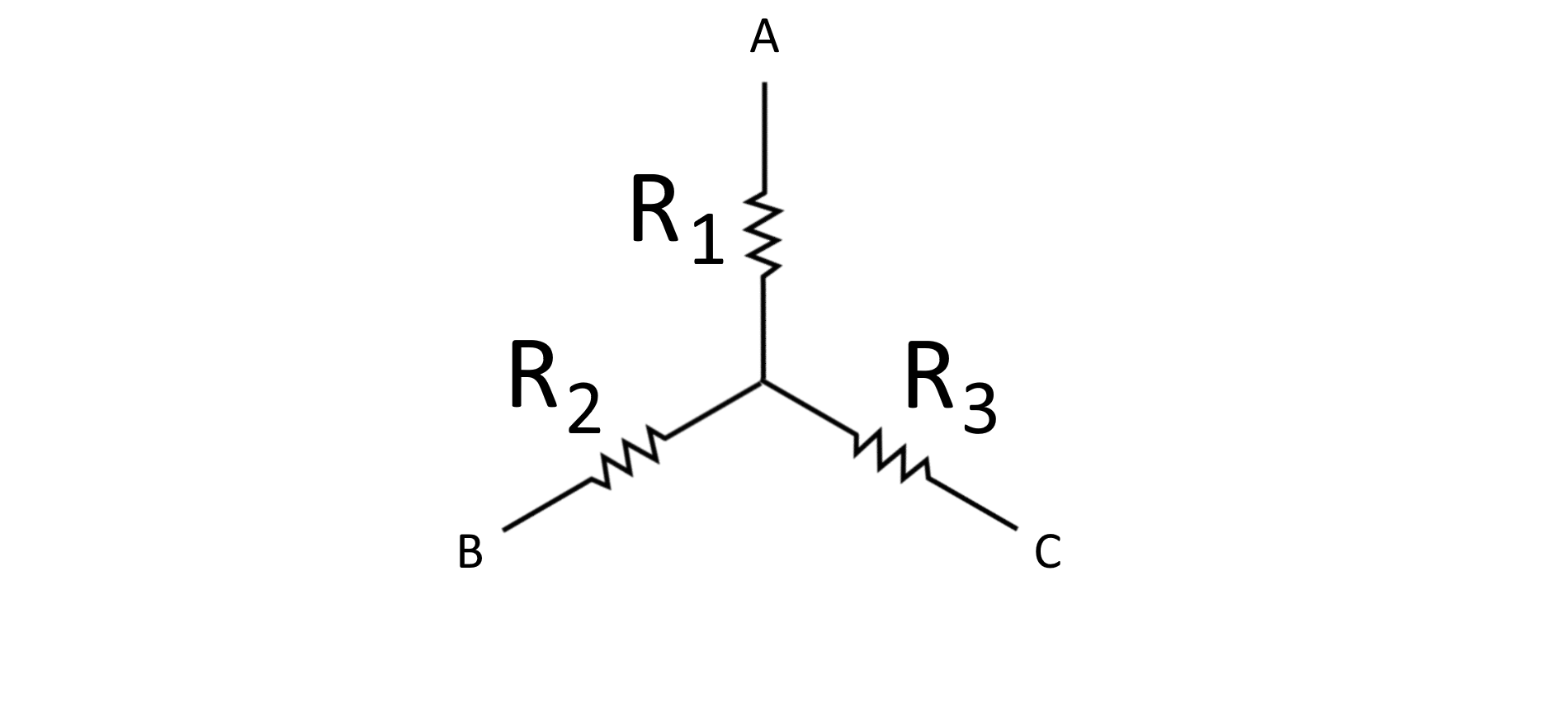To perform a delta to wye conversion, we have to determine the set of resistors to use that will result in an equivalent overall network. The currents and voltages that pass through each of the three nodes must be the same in both types of networks. This is where our delta to wye conversion calculator comes in handy. But if you want to learn how to convert delta to wye networks, and vice versa, yourself, we will show you how it is done in the next section.

## How to convert delta networks to wye networks? And vice versa

Knowing how to determine wye or delta networks in a circuit is the first step in conversion. Converting delta networks to wye networks is a matter of determining the "delta" in a circuit, knowing the resistance values of each resistor in the delta, and using the formulas we included in this text.

The same procedure also applies to the conversion of wye networks to delta networks. However, in this operation, we first have to determine which "wye" needs converting, then find the resistance values in each leg of the wye network. Finally we substitute these values in the formulas provided below.

#### Delta to wye conversion formulas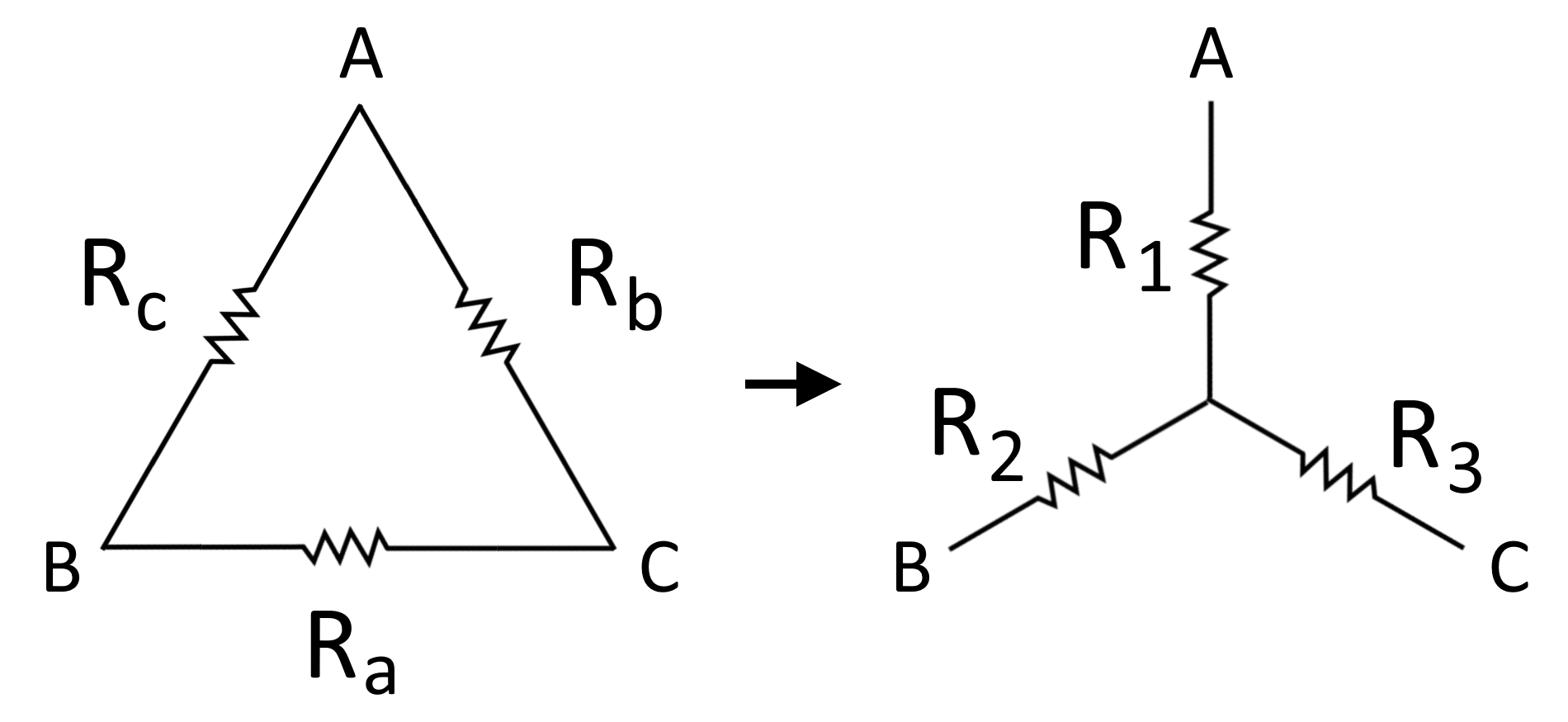R1 = (Rb * Rc)/(Ra + Rb + Rc)

R2 = (Rc * Ra)/(Ra + Rb + Rc)

R3 = (Ra * Rb)/(Ra + Rb + Rc)

where:

• Ra, Rb, Rc are the resistances in the delta network,
• R1, R2, R3 are the resistances in the wye network.

#### Wye to delta conversion formulas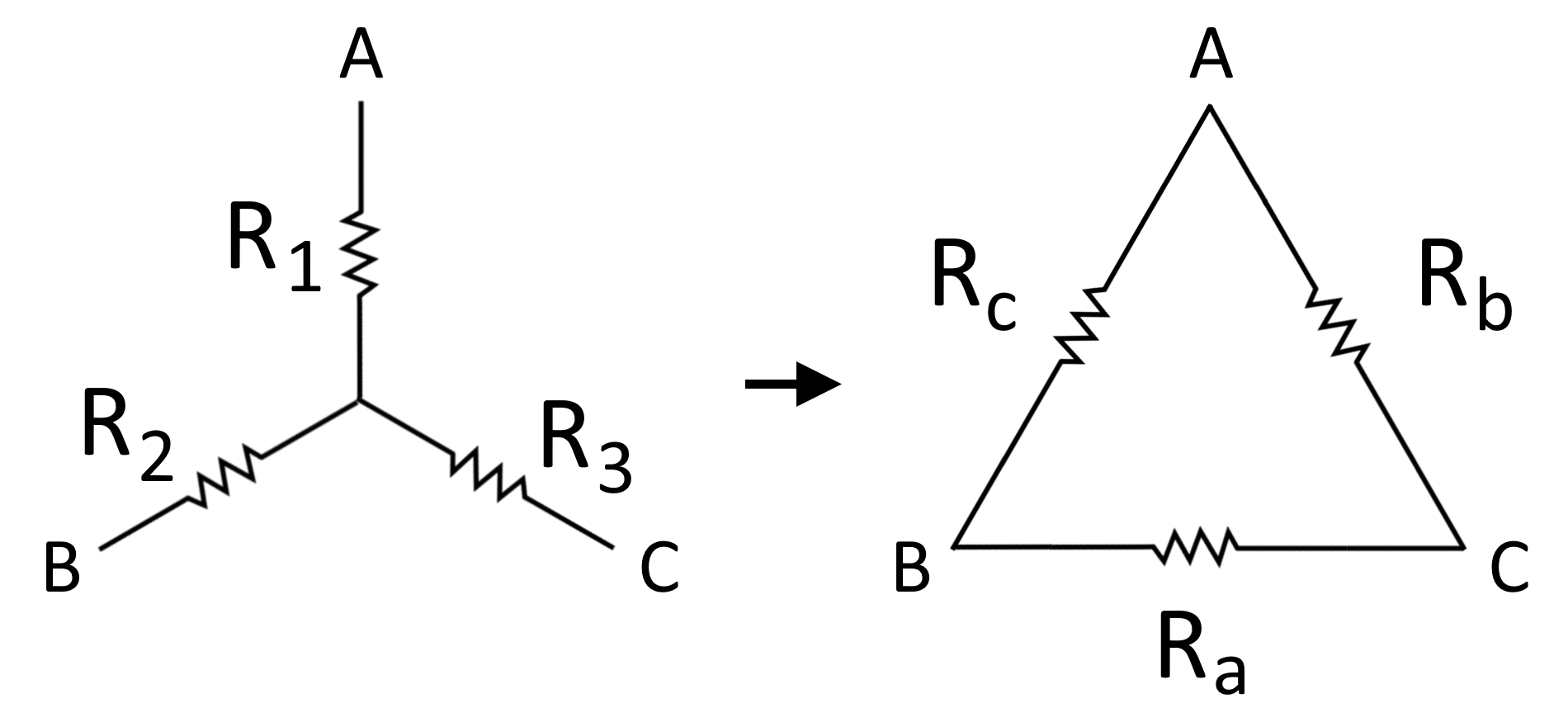Ra = R2 + R3 + (R2 * R3 / R1)

Rb = R3 + R1 + (R3 * R1 / R2)

Rc = R1 + R2 + (R1 * R2 / R3)

where, as previously:

• Ra, Rb, Rc are the resistances in the delta network,
• R1, R2, R3 are the resistances in the wye network.

If you are looking at an actual circuit and you want to know the resistance value of a resistor without using a multimeter, you can tell it by looking at its color bands. You can use our resistor color code calculator to easily determine its corresponding resistance value. On the other hand, to further understand how to use the formulas above, let us consider an example in the next section of this text.

## Delta to Wye Conversion. Sample calculation

For our sample calculation, let us consider the slightly complicated circuit shown below, and convert the delta network in it (we highlighted it in green) to a wye network: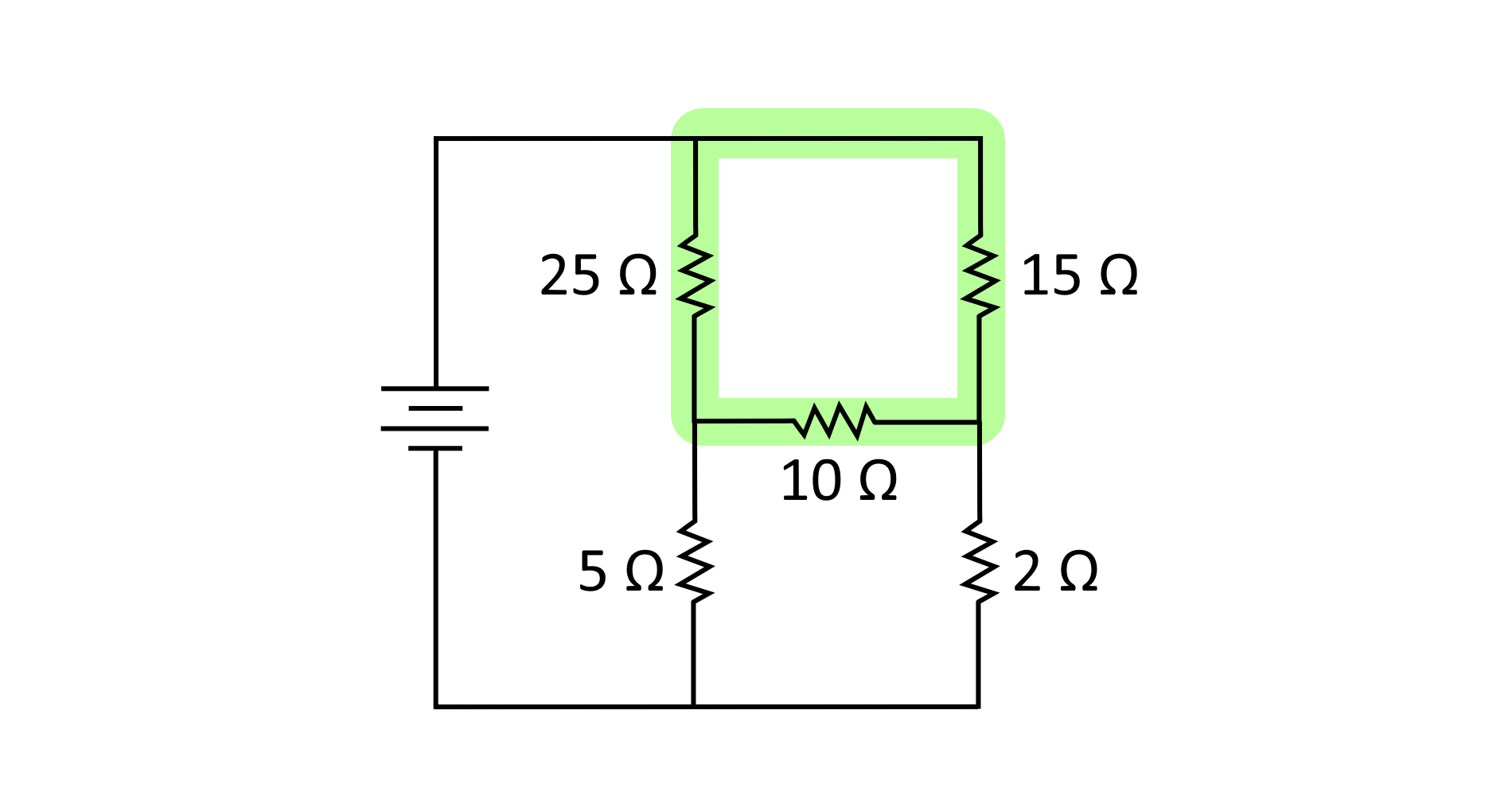The delta diagram might seem different than the ones we showed earlier, but if you look closely, we can actually draw the delta shape, like this: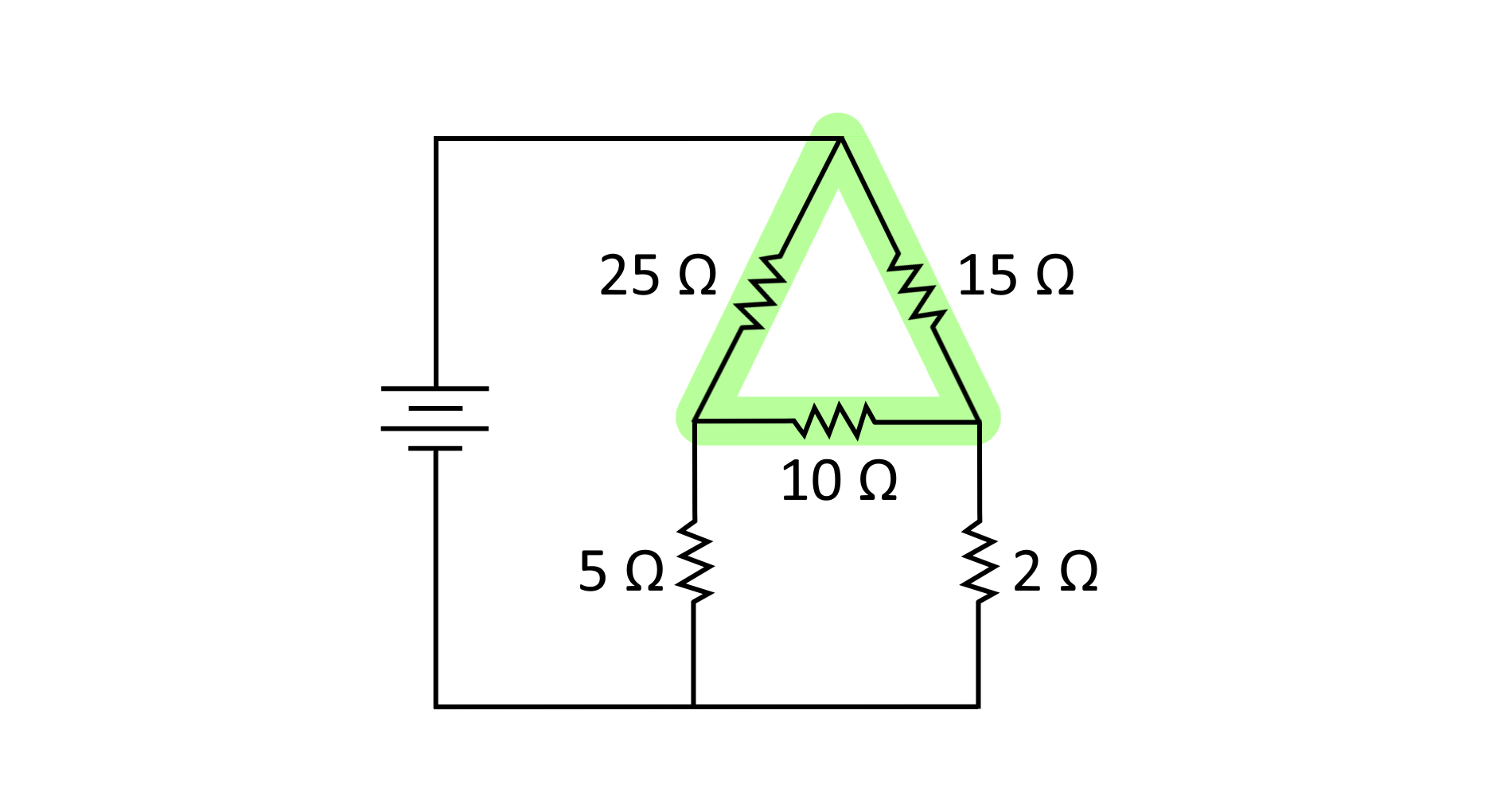Now that we can see the familiar delta diagram in the circuit, let us now draw the corresponding equivalent wye network that our delta network will be transformed into. We can simply draw it over the delta network to preserve the location of the nodes or we can draw the new wye network in a separate equivalent circuit. This is also the best time to label our resistors with the same variables we have in our formulas and reference diagram, as shown below: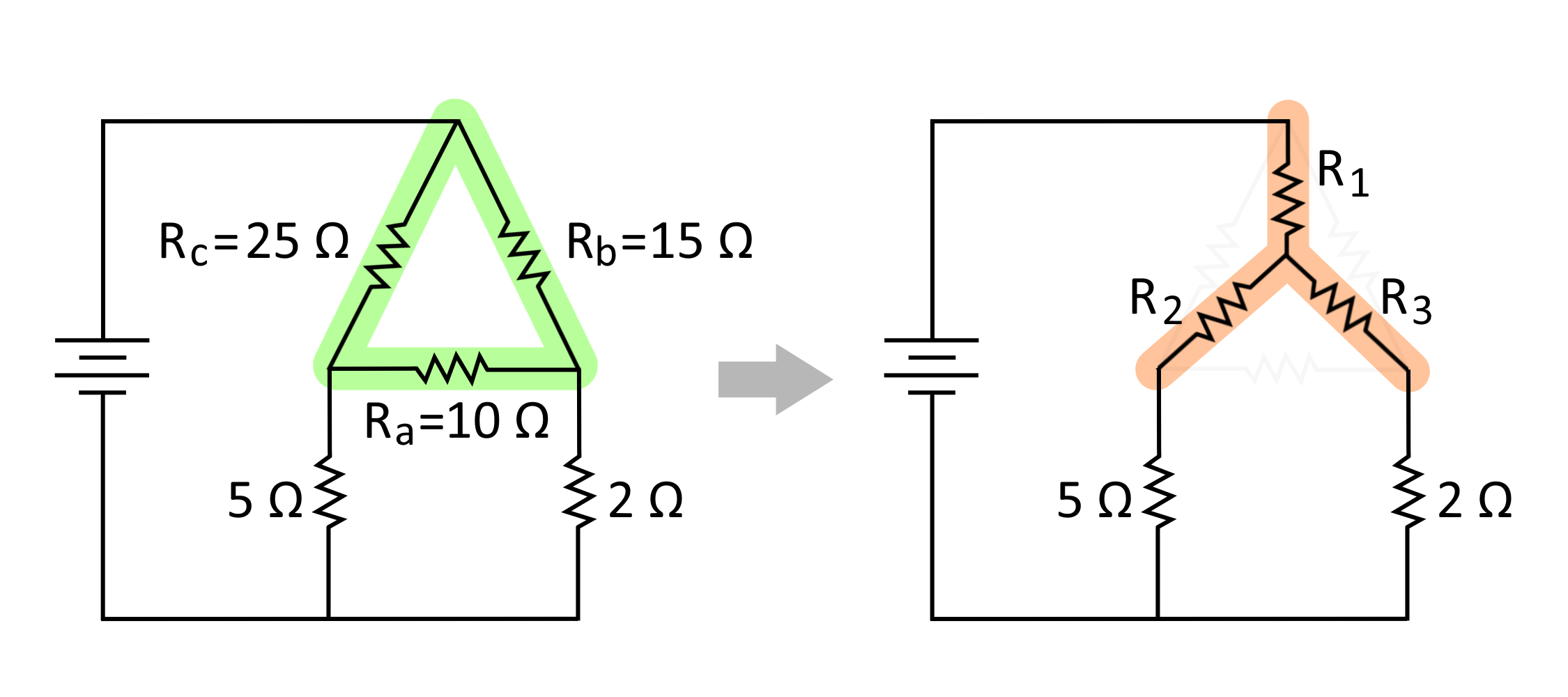Once we have it all set up, we can now use the formulas we have for the delta to wye conversion to find the resistance values for each resistor in the equivalent wye network for the given circuit. Here is the calculation of each resistance values:

R1 = (Rb * Rc)/(Ra + Rb + Rc)

R1 = (15 Ω * 25 Ω)/(10 Ω + 15 Ω + 25 Ω)

R2 = (375 Ω)/(50 Ω)

R1 = 7.5 Ω

R2 = (Rc * Ra)/(Ra + Rb + Rc)

R2 = (25 Ω * 10 Ω)/(10 Ω + 15 Ω + 25 Ω)

R2 = (250 Ω)/(50 Ω)

R2 = 5.0 Ω

R3 = (Ra * Rb)/(Ra + Rb + Rc)

R3 = (10 Ω * 15 Ω)/(10 Ω + 15 Ω + 25 Ω)

R2 = (150 Ω)/(50 Ω)

R3 = 3.0 Ω

From these computations, we can now replace our delta network with a wye network using another set of resistors with resistance values of 7.5 Ω, 5.0 Ω, and 3.0 Ω for R1, R2, and R3, respectively.

## Importance of transforming delta and wye networks

You may have noticed that in the example above, the original network did not have any parallel or series connections for any of the resistors. Instead, we there were two deltas that cannot be simplified without the knowledge of delta to Y conversion.

After conversion, we can now see that R2 resistor = 5 Ω is now in series with the other 5 Ω resistor. In that case, they can now be simplified even further by adding their resistance values together and replacing them with a single 10 Ω resistor. You can learn more about series resistors by checking out the of our series resistor calculator.

With that said, delta to wye conversion, and vice versa, is the perfect method to simplify complicated circuitry when performing circuit analysis. We perform circuit analysis to determine the current or voltage flowing in our electronic circuitand it is best done with a simplified circuit or some parts of it.

If you find this calculator and text useful and informative, perhaps you would find our electricity cost calculator fun and useful too. In there you will learn about how to calculate your electricity cost depending on the power you use at home.

Bogna Szyk and Kenneth Alambra
Operation
Delta to wye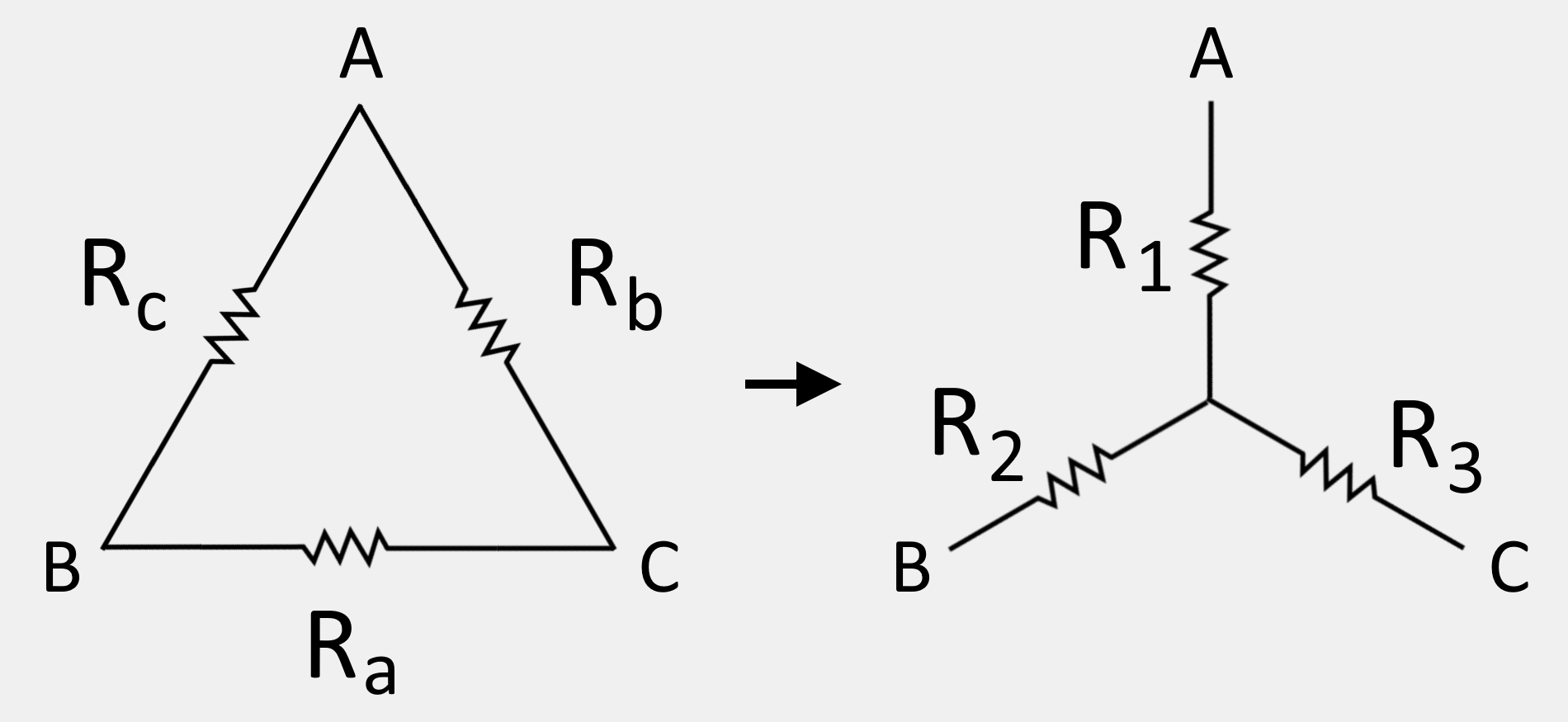Delta to wye conversion
Ra
Ω
Rb
Ω
Rc
Ω
Resulting resistance values
R1
Ω
R2
Ω
R3
Ω
People also viewed…

### Insertion loss

Compute the insertion loss of a two-port network using the insertion loss calculator.

### Plastic Footprint

Find out how much plastic you use throughout a year with this plastic footprint calculator. Rethink your habits, reduce your plastic waste, and make your life a little greener.

### Sleep

The sleep calculator can help you determine when you should go to bed to wake up happy and refreshed.